# Chapter 19 Volume and Surface Area of Solids RS Aggarwal Solutions MCQ Class 10 Maths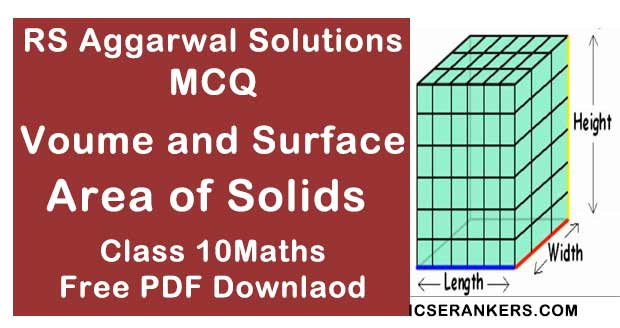Chapter Name RS Aggarwal Chapter 19 Volume and Surface Area of Solids Book Name RS Aggarwal Mathematics for Class 10 Other Exercises Exercise 19AExercise 19BExercise 19CExercise 19D Related Study NCERT Solutions for Class 10 Maths

### Volume and Surface Area of Solids MCQ Solutions

1. A Cylindrical pencil sharpened at one edge is the combination of

A. a cylinder and a cone

B. a cylinder and frustum of a cone

C. a cylinder and a hemisphere

D. Two cylinders

Solution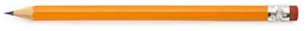Pencil is a combination of cylinder + Cone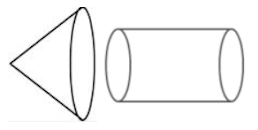2. A shuttlecock used for plating badminton is the combination of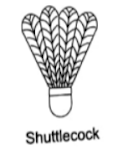A. Cylinder and a hemisphere

B. Frustum of a cone and a hemisphere

C. A cone and a hemisphere

D. A cylinder and a sphere

Solution

Shuttle is a combination of Frustum of a cone + Hemisphere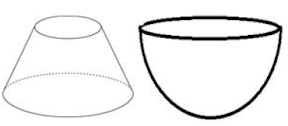3. A funnel is the combination of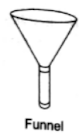A. a cylinder and a cone

B. a cylinder and a hemisphere

C. a cylinder and frustum of a cone

D. a cylinder and a hemisphere

Solution

Funnel is a combination of Frustum of a cone + Cylinder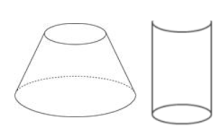4. A Sarahi is a combination of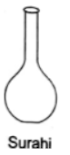A. a sphere and a cylinder

B. a hemisphere and a cylinder

C. a cylinder and a cone

D. Two hemispheres

Solution

Sarahi is a combination of Sphere + Cylinder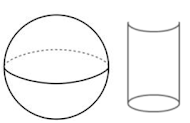5. The shape of a glass (tumbler) is usually in the form of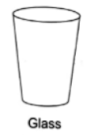A. a cylinder

B. frustum of cone

C. a cone

D. a sphere

Solution

Glass is in the shape of a Frustum of a cone.6. The shape of a Gilli in the Gilli-Danda game is a combination of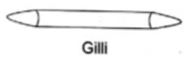A. a cone and a cylinder

B. two cylinder

C. two cones and a cylinder

D. two cylinder and a cone

Solution

Gilli in the gilli-Danda is a combination of Frustum of a frustum of a cone + Cylinder + cone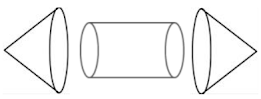7. A plumbline (sahul) is the combination of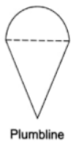A. A hemisphere and a cone

B. A cylinder and a cone

C. A cylinder and a cone

D. A cylinder and frustum of a cone

Solution

Plumbline (sahul is a combination of a cone + hemisphere)

8. A cone is cut by a plane parallel to its base and the upper part is removed. The part that is left over is called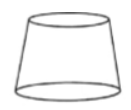A. A cone

B. A sphere

C. A cylinder

D. Frustum of a cone

Solution

When a cone is cut by a plane parallel to its base and the upper part is removed. The part that is left over is called frustum of a cone.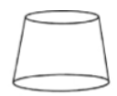9. During conservation of a solid from one shape to another, the volume of the new shape wil

A. Decrease

B. Increase

C. Remain unaltered

D. Be doubled

Solution

When a object of certain volume is melted and converted to some other shape, the volume of the new object formed will be the same as the volume of the old object.

10. In a right circular cone, the cross section made by a plane parallel to the base is a

A. Sphere

B. Hemisphere

C. Circle

D. A semicircle

Solution

In a right circular cone, the cross section made by a plane parallel to the base is a circle.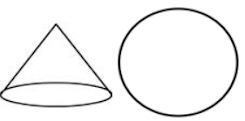11. A solid piece of iron in the form of a cuboid of dimensions (49 cm × 33 cm × 24 cm) is moulded to form a solid sphere. The radius of the sphere is

A. 19 cm

B. 21 cm

C. 23 cm

D. 25 cm

Solution

Given: Dimension of cuboid (49 cm × 33 cm × 24 cm)

Volume of cuboid is: length × breadth × height

Volume of Solid Sphere is 4/3 ×π × r3 (here r is radius of the sphere)

Let v1 be the volume of given cuboid.

∴ v1 = 49 cm × 33 cm × 24 cm = 38808 cm3

Let v2 be the volume of Solid sphere.

We know that when a object is moulded from one shape to other its volume does not change.

∴ v1 = v2

That is,

38808 = 4/3 × π × r3

r3 = 3/4π × 38808

⇒ r3 = 9261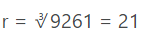∴ r = 21 cm

That is, radius of the solid sphere = 21 cm

12. The radius (in cm) of the largest right circular cone that can be cut out from a cube of edge of 4.2 cm is

A. 2.1

B. 4.2

C. 8.4

D. 1.05

Solution

Given: edge of the cube = 4.2 cm

A right circular cone is a Cone whose height is perpendicular to the diameter (radius) of the base circle.

In a cube, a largest right circular cone is formed when its base lies on one of the faces of the cube and its tip lies on the opposite face.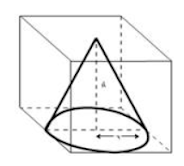∴ Diameter of largest right circular Cone in cube = edge length of cube.

∴ Diameter = 4.2 cm

⇒ Radius = diameter/2 = 4.2.2 = 2.1 cm

∴ Radius of the largest right circular cone is cube is 2.1 cm

13. A metallic solid sphere of radius 9 cm is melted to form a solid cylinder of radius 9 cm. The height of the cylinder is

A. 12 cm

B. 18 cm

C. 36 cm

D. 96 cm

Solution

Given:

Radius of the solid sphere = 9 cm

Radius of the cylinder = 9 cm

Volume of Solid Sphere is 4/3 × π × r3 (here r is radius of the sphere)

Volume of Solid Cylinder is : π × r2 × h (here r is radius and h is height of the cylinder)

Let v1 be the volume of given Solid sphere.

∴ v1 = 4/3 × π × 93 cm3

Let v2 be the volume of Solid Cylinder.

∴ v2 = π × 92 × h

We know that when a object is moulded from one shape to other its volume does not change.

∴ v1 = v2

That is,

4/3 × π × 93 = π × 92 × h

⇒ h = (4/3 × π × 9 × 9 × 9)/(π × 9 × 9) = 4/3 × 9

= 12 cm

∴ h = 12 cm

That is Height of the Cylinder is 12 cm

14. A rectangular sheet of paper 40 cm × 22 cm, is rolled to form a hollow cylinder of height 40 cm. The radius of the cylinder (in cm) is

A. 3.5

B. 7

C. 80/7

D. 5

Solution

Given:

Dimensions of rectangular sheet = 40 cm × 22 cm

Height of the Hollow Cylinder : 40 cm

Area of the Rectangle is = length × breadth

Curved surface area of the Cylinder = 2πrh (where r and h area radius and height of cylinder respectively)

Let a1 be the area of Rectangle

a1 = 40 × 22 cm2

Let a2 be the curved surface area of cylinder

a2 = 2 × π × r × 40 cm2

We know that when the area of a surface doesn’t change even if its shape is changed.

a1 = a2

40 × 22 = 2 × π × r × 40

r = (40 × 22)/(2 × π × 40) = 11/π = (11 × 7)/22 = 7/2 = 3.5 cm

Radius of the Cylinder is 3.5 cm.

15. The number of solid sphere, each of diameter 6 cm, that can be made by melting a solid metal cylinder of height 45 cm and diameter 4 cm, is

A. 2

B. 4

C. 5

D. 6

Solution

Given: Diameter of the Solid Sphere is 6 cm

Height of the Cylinder is 45 cm

Diameter of the Cylinder is 4 cm

Volume of Solid Cylinder is : π × r2 × h (here r is radius and h is height)

Volume of the Solid Sphere is : 4/3 × π × r3 (here r is the radius of the sphere)

Let v1 be the volume of given cylinder

v1 = π × 22 × 45 cm3 (4 cm is diameter, 2 cm is the radius of cylinder)

Let v2 be the volume of Solid sphere.

V2 = 4/3 × π × 33 cm3 (6 cm is the diameter, 3 cm is the radius of the sphere)

We know that when a object is moulded from one shape to other its volume does not change.

Let n be the number of solid Sphere of diameter 6 cm required.

v1 = n × v2 (volume of n Spheres = Volume of cylinder)

That is,

π × 22 × 45 = n × 4/3 × π × 33

⇒ n = (π × 2 × 2 × 45)/(4/3 × π × 3 × 3 × 3)

⇒ n = (2 × 2 × 45 × 3)/(4 × 3 × 3 × 3)

⇒ n = 45/9 = 5

That is 5 Solid Spheres of diameter 6 cm can be formed by the Solid cylinder of height 45 cm and diameter 4 cm.

16. The surface areas of two sphere are in the ratio 16: 9. The ratio of their volume is

A. 64 : 27

B. 16 : 9

C. 4 : 3

D. 163 : 93

Solution

Given:

Surface area ratio of two spheres is 16 : 9

Volume of the sphere is 4/3 × π × r3 (where r is radius of sphere)

Surface area of the sphere is 4 × π × r2 (Where r is radius of sphere)

Let S1 and S2 be two different spheres.

(Surface area of) S1 : (Surface area of) S2 = 16 : 9

4 × π × (r1)2 : 4 × π × (r2)2 = 16 : 9 (here r1 and r2 are the radii of S1 and S2 respectively)

(r1)2 : (r2)2 = 16 : 9

⇒ r1 : r2 = √16 : √9

⇒ r1 : r2 = 4 : 3

Now,

Let V1 and V2 be the volumes of the spheres S1 and S2 respectively.

∴ V1 : V2 = 4/3 × π × (r1)3 : 4/3 × π ×(r2)3 (here r1 and r2 are the radii of S1 and S2 respectively)

⇒ V1 : V2 = (r1)3 : (r2)3

⇒ V1 : V2 = (4)3 : (3)3

⇒ V1 : V2 = 64 : 27

∴ The ratios of the volumes is 64 : 27

17. If the surface area of a sphere is 616 cm2, its diameter (in cm) is

A. 7

B. 14

C. 28.

D. 56

Solution

Given: Surface area of a sphere is 616 cm2

Surface area of the sphere is : 4 × π × r2 (where r is radius of sphere)

∴ 4 × π × r2 = 616 cm2

⇒ r2 = 616/(4 × π) = 154/π = 49

⇒ r = √49 = 7 cm

∴ Diameter = 2 × r = 2 × 7 = 14 cm

18. If the radius of a sphere becomes 3 times then its volume will become

A. 3 times

B. 6 times

C. 9 times

D. 27 times

Solution

Let r1 be the initial radius of the sphere.

∴ r1 = r

Let r2 be the radius after increasing it 3 times the size of initial radius.

∴ r2 = 3r

Let V1 be the initial volume of the sphere

∴ V1 = 4/3 × π × (r1)3 = 4/3 × π × r3

Let V2 be the volume of the sphere after its radius is increased by 3 times.

∴ V2 = 4/3 × π × (r2)3 = 4/3 × π × (3r)3

⇒ V2 = 27 × 4/3 × π × r3

∴ If radius is increased by 3 times, its volume will be increased by 27 times.

19. If the height of a bucket in the shape of frustum of a cone is 16 cm and the diameter of its two circular ends are 40 cm and 16 cm then its slant height is

A. 20 cm

B. 12√5 cm

C. 8√13

D. 16 cm

Solution

Given: Height of the frustum of a cone : 16 cm

Diameters of the Circular ends: 40 cm and 16 cm.

Radius of the Circular ends: 40/2 = 20 cm and 16/2 = 8 cm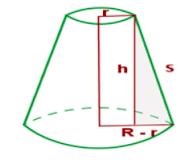Here slant height h can be found by using Pythagoras theorem.

∴ s2 = h2 + (R - r)2 (here R is 20 cm and r is 8 cm)

⇒ s2 = 162 + (20 – 8)2

⇒ s2 = 162 + (12)2

⇒ s2 = 256 + 144

⇒ s2 = 400

⇒ s = √400 = 20

∴ Slant height of the frustum is 20 cm.

20. A sphere of diameter 18 cm is dropped into a cylinder vessel of diameter 36 cm, partly filled with water. If the sphere is completely submerged then the water level rises by

A. 3 cm

B. 4 cm

C. 5 cm

D. 6 cm

Solution

Given: Diameter of a sphere : 18 cm

⇒ radius = 18/2 = 9 cm

Diameter of Cylindrical vessel : 36 cm

⇒ radius = 36/2 = 18 cm

It is given that Sphere is dropped into the cylindrical vessel containing some water.

∴ Volume of sphere = Volume of water in Cylinder displaced (raised)

Let V1 be the volume of the sphere

∴ V1 = 4/3 × π × (r1)3

⇒ V1 = 4/3 × π × 93

Let V2 be the volume of the water displaced in the cylindrical vessel

∴ V2 = π × (r2)2 × h (here r2 is the radius of the cylinder and h is the level of water raised in the vessel after dropping the sphere into the cylindrical vessel)

V2 = π × 182 × h

Since V1 = V2

4/3 × π × 93 = π × 182 × h

⇒ h = (4/3 × π × 9 × 9 × 9)/(π × 18 × 18)

⇒ h = (4 × 9 × 9 × 9)/(3 × 18 × 18) = 3 cm

∴ The water level rises by 3 cm when the dropped sphere is completely submerged in the cylindrical vessel.

21. A solid right circular cone is cut into two parts at the middle of its height by a plane parallel to its base. The ratio of the volume of the smaller cone to the whole cone is

A. 1 : 2

B. 1 : 4

C. 1 : 6

D. 1 : 8

Solution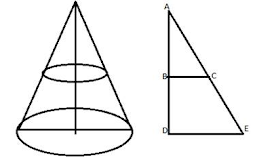Given: A solid right circular cone is cut into two parts at the middle of its height by a plane parallel to its base

Let ‘H’ be the height of the cone.

Let ‘R’ be the Radius of the complete cone.

Volume of a cone is given by 1/3πr2h

Here,

AB = BD = H/2

Let r be the radius of the smaller cone.

∠CAB = ∠EAB (common)

∴ △ABC ~ △ADE (AA similarity criterion)

⇒ OA/OC = AB/CD (Corresponding sides are proportional)

⇒ H/(H/2) = R/r

⇒ R = 2r

Volume of smaller cone = 1/3π(r)2 × h = 1/3π(BC)2 × AB = 1/3π(r)2 × H/2 = (π × r × H)/6 cm3

Volume of whole cone = 1/3π(r)2 × h = 1/3π(DE)2 × AD = 1/3π(2r)2 × H = 4/3πr2H cm3

∴ (Volume of smaller cone)/(Volume of whole cone) = {(π × r × H)/6}/(4/3.π × r × r × H) = 1/8

∴ The ratio of the volume of the smaller cone to the whole cone is 1 : 8

22. The radii of the circular ends of a bucket of height 40 cm are 24 cm and 15 cm. The slant height (in cm) of the bucket is

A. 41

B. 43

C. 49

D. 51

Solution

Bucket is in the shape of a frustum of a cone

Therefore,

Given: height of the frustum : 40 cm

Radius of the Circular ends : 24 cm and 15 cm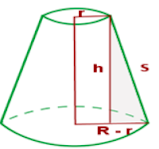Here slant height h can be found by using Pythagoras theorem.

∴ s2 = h2 + (R – r)2 (here R is 20 cm and r is 8 cm)

⇒ s2 = 402 + (24 – 15)2

⇒ s2 = 402 + (9)2

⇒ s2 = 1600 + 81

⇒ s2 = 1681

⇒ s = √1681 = 41

∴ Slant height of the Frustum is 41 cm

23. A solid is hemispherical at the bottom and conical (of same radius) above it. If the surface areas of the two parts area equal then the ratio of its radius and the slant the height of the conical part is

A. 1 : 2

B. 2 : 1

C. 1 : 4

D. 4 : 1

Solution

Given:

Bottom of a solid is hemispherical and conical above it, both have same radius

And same surface areas.

∴ CSA of hemisphere = CSA of Cone

⇒ 2 × π × r2 = πrl (where r is the radius and l is the slant height)

⇒ (r × r)/(r × 1) = π/(2 × π)

⇒ r/1 = 1/2

∴ r : l = 1 : 2

That is ratio of radius and the slant height of the given solid is 1 : 2.

24. If the radius of the base of a right circular cylinder is halved, keeping the height the same. Then the ratio of the volume of the cylinder thus obtained to the volume of original is

A. 1 : 2

B. 2 : 1

C 1 : 4

D. 4 : 1

Solution

Given : radius of the base of a right circular cylinder is halved, keeping the height the same.

Let initial radius Circular Cylinder be ‘r’.

∴ Radius of the Cylinder after its radius is halved is ‘r/2’

Let ‘h’ be the height of the both the cylinders.

Let V1 be the volume of the initial Cylinder.

Let V2 be the volume of the cylinder after the initial Cylinders base radius is halved.

Volume of the Cylinder is given by : πr2h

∴ V1 : V2 = (πr1)2h: π(r2)2h

∴ V1: V2 = π(r)2h : π(r/2)2h

⇒ V1 : V2 = 1 : 1/4

⇒ V1 : V2 = 4 : 1

Thus, ratio of the volume of the cylinder thus obtained to the volume of original is 4 : 1.

25. A cubical ice-cream brick of edge 22 cm is to be distributed among some children by filled ice-cream cones of radius 2 cm and height 7 cm upto its brim. How many children will get the ice-cream cones ?

A. 163

B. 263

C. 363

D. 463

Solution

Given : Cubical ice-cream brick of edge 22.

Ice-cream cone of radius 2 cm and height 7 cm.

Let ‘n’ be the number of students who get ice-cream cones.

Let V1 be the volumes of the Cubical ice-cream brick.

Volume of Cube is given by a3 : (where a is the edge length)

∴ V1 = a3 = 223

Let V2 be the Volume of the Ice-cream Cone.

Volume of cone is given by: 1/3 × π × r2 × h (where r is the radius of the base and h is the edge height of the cone)

∴  V2 = 1/3 × π × r2 × h = 1/3 × π × 22 × 7

Here,

V1 = n × V2

∴ 223 = n × 1/3 × π × 22 × 7

⇒ n = (22 × 22 × 22 × 3)/(π × 2 × 2 × 7)

⇒ n = (22 × 22 × 22 × 3 × 7)/(22 × 2 × 2 × 7)

⇒ n = 363

∴ 363 Children can get ice cream cones.

26. A mason constructs a wall of dimension (270 cm × 300 cm × 350 cm) with bricks, each of size (22.5 cm × 11.25 cm × 8.75 cm) and it is assumed that 1/8 space is covered by the mortar. Number of bricks used to construct the wall is

A. 11000

B. 11100

C. 11200

D. 11300

Solution

Given :

A wall of dimension (270 cm × 300 cm × 350 cm)

A wall of dimension (22.5 cm × 11.25 cm × 8.75 cm).

1/8 space is covered by the mortar.

Here 1/8 volume of the wall is covered by mortar, ∴ 1 – 1/8 = 7/8

Volume of the wall is covered by bricks.

Volume of the cuboid is given by: lbh (here l, b, h are length, breadth, height respectively).

Let V1 be the volume of the wall.

∴ V1 = l × b × h = 270 × 300 × 350

⇒ V1 = 270 × 300 × 350 = 28350000 cm2

Let V2 be the volume of the brick.

∴ V2 = l × b × h = 22.5 × 11.25 × 8.75

⇒ V2 = 22.5 × 11.25 × 8.75 = 70875/32 cm2

Let ‘n’ be the number of bricks required to occupy 7/8 volume of the wall.

∴ n × 70875/32 = 28350000 × 7/8

⇒ n = 28350000 × 7/8 × 32/70875 = 11200

∴ n = 11200

That is 11200 bricks required to occupy 7/8 volume of the wall.

27. Twelve solid sphere of the same size are made by melting a solid metallic cylinder of base diameter 2 cm and height 16 cm. The diameter of each sphere is

A. 2 cm

B. 3 cm

C. 4 cm

D. 6 cm

Solution

Given : A solid metallic cylinder of base diameter 2 cm and height 16 cm.

Twelve solid sphere of the same size are made by melting a solid metallic cylinder.

Let V1 be the volume of the cylinder.

Volume of the cylinder s given by : π × r2 × h

∴ V1 = π × 12 × 16 (diameter is 2 cm, ∴ radius is 1 cm)

Let V2 be the volume of the each sphere.

Volume of the Sphere is given by: 4/3 × π × r3 (where r is the radius of sphere)

∴ V2 = 4/3 × π × r3

Here,

V1 = 12 × V2

∴ π × 12 × 16 = 12 × 4/3 × π × r3

⇒ r3 = (3 × π × 16)/(π × 4 × 12) = (π × 16)/(π × 4 × 4) = 1 cm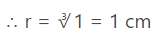∴ Diameter of the sphere is 2 × r = 2 × 1 = 2 cm

28. The diameter of two circular ends of a bucket area 44 cm and 24 cm, and the height of the bucket is 35 cm. The capacity of the bucket is

A. 31.7 litres

B. 32.7 litres

C. 33.7 litres

D. 34.7 litres

Solution

Given: diameter of two circular ends of a bucket area 44 cm and 24 cm, and the height of the bucket is 35 cm.

Bucket is in the shape of frustum.

Let V be the Volume of the Bucket (Frustum)

Volume of the frustum is given by : π/3 × h × (R2 + r2 + Rr) (here r and R are the radii of smaller and larger circular ends respectively)

∴ V = π/3 × h × (R2 + r2 + R × r)

⇒ V = π/3 × 35 × (222 + 122 + 22 × 12) (diameters are 44 and 24 cm, ∴ their radii are 22 cm and 12 cm respectively)

⇒ V = π/3 × 35 × (484 + 144 + 264) = π/3 × 35 × (892)

⇒ V = π/3 × 35 × (892) = 32706.6 cm3 = 32.7 litres (∵ 1000 cm3 = 1 litre)

∴ The capacity of the bucket is 32 : 7 litres

29. The slant height of a bucket is 45 cm and the radii of its top and bottom are 28 cm and 7 cm respectively. The curved surface area of the bucket is

A. 4953 cm2

B. 4952 cm2

C. 4951 cm2

D. 4950 cm2

Solution

Given : Slant height of a bucket is 45.

Radii of its top and bottom are 28 cm and 7 cm respectively.

Bucket is in the shape of a frustum.

Let A be the CSA of the frustum

CSA of the frustum is given by: πl(r1 + r2) (here l is the slant height and r1 and r2 are the radii of top and bottom of the bucket (frustum))

∴ A = πl(r1 + r2)

⇒ A = π × 45 × (28 + 7) = π × 45 × (35) = 4950 cm2

∴ A = 4950 cm2

That is curved surface area of the bucket is 4950 cm2

30. The volumes of two spheres are in the ratio 64 : 27. The ratio of their surface areas is

A. 9 : 16

B. 16 : 9

C. 3 : 4

D. 4 : 3

Solution

Given : Volume ratio of two spheres is 64 : 27

Volume of the Sphere is: 4/3 × π × r3 (where r is radius of sphere)

Surface area of the sphere is: 4 × π × r2 (where r is radius of sphere)

Let S1 and S2 be two different spheres.

(Volume of) S1 : (Volume of) S2 = 64 : 27

4/3 × π × (r1)3 : 4/3 × π × (r2)3 = 64.27 (here r1 and r2 are the radii of S1 and S2 respectively)

⇒ (r1)3 : (r2)3 = 64 : 27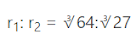⇒ r1 : r2 = 4 : 3

Now,

Let SA1 and SA2  be the surface areas of the spheres S1 and S2 respectively.

∴ SA1 : SA2 = 4 × π × (r1)2 : 4 × π × (r2)2 (here r1 and r2 are the radii of S1 and S2 respectively)

⇒ SA1 : SA2 = (r1)2 : (r2)2

⇒ SA1 : SA2 = (4)2 : (3)2

⇒ SA1 : SA2 = 16 : 9

∴ The ratio of the Surface area of spheres is 16 : 9.

31. A hollow cube of internal edge 22 cm is filled with spherical marbles of diameter 0.5 cm and 1/8 space of the cube remains unfilled. Number of marbles required is

A. 142296

B. 142396

C. 142496

D. 142596

Solution

Given: hollow cube of internal edge 22 cm

Spherical marbles of diameter 0.5 cm

⇒ radius = 0.5/2 = 0.25 cm

1/8 space of the cube remains unfilled.

Here, 1/8 space of the cube is unfilled, that is 7/8 of the cube is filled with marbles of radius 0.25 cm.

Volume of the sphere is : 4/3 × π × r3 (where ‘r’ is the radius of the cube)

Let V1 be the volume of the Sphere.

∴ V1 = 4/3 × π × (0.25)3 = 11/168 cm3

Volume of the Cube : a3 (where ‘a’ is the edge length of the cube)

Let V2 be the volume of the cube.

∴ V1 = (22)3 = 10648 cm3

Let ‘n’ be the number of sphere required to fill the 7/8 space of the cube.

∴ n × V1 = V2 × 7/8

⇒ n × 11/168 = 10648 × 7/8

⇒ n × 11/168 = 9317

⇒ n = 9317 × 168/11 = 142296

∴ Number of marbles required to fill 7/8 of the cuboid 142296.

32. A metallic spherical shell of internal and external diameter 4 cm and 8 cm respectively, is melted and recast into the form of a cone of the base diameter 8 cm. The height of the cone is

A. 12 cm

B. 14 cm

C. 15 cm

D. 8 cm

Solution

Given: Metallic spherical shell of internal and external diameter 4 cm and 8 cm respectively.

Recast into the form of a cone of base diameter 8 cm.

Volume of spherical shell is: 4/3 × π × [(r1)3 – (r2)3] (here r1 and r2 are external and internal radii respectively)

Let v1 be the volume of given Spherical shell.

∴ v1 = 4/3 × π × [(8/2)3 – (4/2)3] = 4/3 × π × [(4)3 – (2)3] = 4/3 × π × [64 – 8] = 4/3 × π × 56 cm3

Volume of the cone is given by: 1/3 × π × r2 × h

Let v2 be the volume of cone.

∴ V2 = 1/3 × π × (8/2)2 × h = 1/3 × π × 16 × h cm3

We know that when a object is moulded from one shape to other its volume does not change.

∴ v1 = v2

That is,

4/3 × π × 56 = 1/3 × π × 16 × h

⇒ h = (4/3 × π × 56)/(1/3 × π × 16)

⇒ h = (4 × 56)/16 = 14

∴ h = 14 cm

That is, height of the cone = 14 cm

33. A medicine capsule is in the shape of a cylinder of diameter 0.5 cm with two hemisphere struck to each of its ends. The length of the entire capsule is 2 cm. The capacity of the capsule is

A. 0.33 cm3

B. 0.34 cm3

C. 0.35 cm3

D. 0.36 cm3

Solution

Given: A cylinder of diameter 0.5 cm

The length of the entire capsule is 2 cm

Here, radius of the Cylinder and Hemisphere is 0.5/2 = 0.25 cm

Height of the cylinder = Length of capsule – 2 × (radius of hemisphere) = 2 – 0.5 = 1.5 cm

Volume of cylinder is πr2h (here r, h are radius and height of the cylinder respectively)

Volume of hemisphere is 4/3πr3 (here r is the radius of the hemisphere)

Let V1 be the Cylindrical part of the capsule

∴ V1 = πr2h = π × (0.25)2 × (1.5) = 0.09375π cm3

Let V2 be the Cylindrical part of the capsule

∴ V1 = 4/3πr3 = 4/3π(0.25)3 = (0.0625)/3.π cm3

∴ Volume of the capsule = V1 + 2V2 = 0.09375π + 2 × 0.0625/3.π

= 0.3599 ≈ 0.36 cm3

∴ Volume of the capsule is 0.36 cm3.

34. The length of the longest pole that can be kept in a room (12 m × 9 m × 8 m) is

A. 29 m

B. 21 m

C. 19 m

D. 17 m

Solution

Given : A room with dimensions (12 m × 9 m × 8 m)

Room is in the shape of a cuboid.

Longest rod that can be placed in a room is nothing but its diagonal.

Let length of the rod be ‘L’.

Length of diagonal of a cuboid = √(l2 + b2 + h2)

∴ L = √(l2 + b2 + h2)

⇒ L = √(122 + 92 + 82)m

⇒ L = √(144 + 81 + 64) m

⇒ L = √289 m

⇒ L = 17 m

∴ The length of the longest rod is 17 m.

35. The length of the diagonal of a cube is 6√3 cm. Its total surface area is

A. 144 cm2

B. 216 cm2

C. 180 cm2

D. 108 cm2

Solution

Given: Length of the diagonal of a cube is 6√3 cm.

We know that the Length of a diagonal of a cube is given by : a√3 (here ‘a’ is edge length of cube)

∴ a√3 = 6√3

⇒ a = 6 cm

∴ Edge length of the cube is 6 cm.

Surface area of a cube is given by : 6a2 (here ‘a’ is the edge length)

Let ‘S’ be the surface area of the given cube.

∴ S = 6a2

⇒ S = 6 × (6)2

⇒ S = 216 cm2

∴ Surface area of the given cube is 216 cm2

36. The volume of a cube is 2744 cm3. Its surface area is

A. 196 cm2

B. 1176 cm2

C. 784 cm2

D.588 cm2

Solution

Given: Volume of a cube is 2744 cm3.

We know that the Volume of a cube is given by : a3 (here ‘a’ is edge length of cube)

∴ a3 = 2744

⇒ a = 14 cm

∴ Edge length of the cube is 14 cm.

Surface area of a cube is given by : 6a2 (here ‘a’ is the edge length)

Let ‘S’ be the surface area of given cube.

∴ S = 6a2

⇒ S = 6 × (14)2

⇒ S = 1176 cm2

∴ Surface area of the given cube is 1176 cm2.

37. The total surface area of a cube is 864 cm2. Its volume is

A. 3456 cm3

B. 432 cm3

C. 1728 cm3

D. 3456 cm3

Solution

Given: The total surface area of a cube is 864 cm2.

We know that the volume of a cube is given by : 6a2 (here ’a’ is edge length of cube)

∴ 6a2 = 864

⇒ a2 = 864/6 = 144

⇒ a = √144

⇒ a = 12 cm

∴ Edge length of the cube is 12 cm.

Volume of a cube is given by : a3 (here ‘a’ is the edge length)

Let ‘V’ be the volume of the given cube.

∴ V = a3

⇒ V = (12)3

⇒ V = 1728 cm3

∴ Volume of the given cube is 1728 cm3

38. How many bricks each measuring (25 cm × 11.25 cm × 6 cm) will be required to construct a wall (8 m × 6 m × 22.5 cm)?

A. 8000

B. 6400

C. 4800

D. 7200

Solution

Given: Bricks each measuring (25 cm × 11.25 cm × 6 cm)

A wall with dimensions (8 m × 6 m × 22.5 cm)

We know that the Brick and wall are in the shape of a cuboid.

∴ Volume of a Brick and wall is given by: l × b × h (here l, b, h are the length, breadth, height of the wall and brick)

Let V1 be the volume of the wall.

∴ V1 = l × b × h = 800 × 600 × 22.5 (∵ 8 m = 800 cm and 6 m = 600 cm)

⇒ V1 = 10800000 cm3

Let V2 be the volume of a Brick.

∴ V2 = l × b × h = 25 × 11.25 × 6

⇒ V2 = 1687.5 cm3

Let ‘n’ be the number of Bricks required to build the wall.

∴ V1 = n × V2

10800000 = n × 1687.5

⇒ n = 10800000/1687.5 = 6400

∴ n = 6400

That is, 6400 bricks are required to build the wall.

39. The area of the base of a rectangular tank is 6500 cm2 and the volume of water contained in it is 2.6 m3. The depth of water in the tank is

A. 3.5 m

B. 4 m

C. 5 m

D. 8 m

Solution

Given:

The area of the base of a rectangular tank is 6500 cm2.

Volume of water contained in it is 2.6 m3.

We know that Rectangular tank is in the shape of a cuboid.

And also area of the base of a cuboid is given by: l × b (here l and b are length and breadth respectively)

∴ l × b = 6500 cm2 …(i)

Volume of a cuboid is given by: l × b × h (here l, b, h are length, breadth, height respectively)

∴ l × b × h = 2.6 m3 = 2.6 × 1000000 cm3 = 2600000 cm3 ...(ii)

From (i) and (ii)

6500 × h = 2600000

H = 2600000/6500 = 400 cm = 400/100 m = 4 m

∴ h = 4 m

That is, depth of water in the tank is 4 m.

40. The volume of a wall, 5 times as high as it is abroad and 8 times as long as it is high, is 12.8 m3. The breadth of the wall is

A. 30 cm

B. 40 cm

C. 22.5 cm

D. 25 cm

Solution

Given: The volume of a wall is 12.8 m3.

Height is 5 times the breadth and length is 8 times the height.

∴ Height = 5x

⇒ Length = 8 × (5x) = 40x

Volume of a cuboid is given by: l × b × h (here l, b, h are length, breadth, height respectively)

∴ l × b × h = 12.8

⇒ x × 5x × 40x = 12.8

⇒ 200x3 = 12.8

⇒ x3 = 12.8/200 m3 = 8/125

∴ x = 0.4 m = 0.4 × 100 = 40 m

That is Breadth is: 40 cm

41. If the areas of three adjacent faces of a cuboid are x, y, z respectively then the volume of the cuboid is

A. xyz

B. 2xyz

C. √xyz

D. 3√xyz

Solution

Given : Areas of three adjacent faces of a cuboid are x, y, z respectively.

Let l, b, h be the length, breadth, height of the cuboid respectively.

∴ l × b = x – 1

b × h = y – 2

h × l = z – 3

Volume of the cuboid is: l × b × h

Multiply eq’s -1, -2, -3

That is,

l × b × b × h × l × h = x × y × x = l2 × b2 × h2

⇒ l2 × b2 × h2 = xyz

⇒ (l × b × h)2 = xyz

⇒ (V)2 = xyz (∵ Volume of the cuboid is l × b × h)

⇒ V = √xyz

∴ V = √xys

That is volume of the given cuboid is √xyz

42. The sum of length, breadth and height of a cuboid is 19 cm and its diagonal is 5√5 cm. Its surface area id

A. 361 cm2

B. 125 cm2

C.236 cm2

D.486 cm2

Solution

Given: Sum of length, breadth and height of a cuboid is 19 cm.

Length of diagonal is 5√5 cm.

Let l, b, h be the length, breadth, height of the cuboid respectively.

∴ l + b + h = 19 cm -1

Length of a diagonal in a cuboid is given by : √(l2 + b2 + h2)

∴ √(l2 + b2 + h2) = 5√5 cm ⇒ (l2 + b2 + h2) = (5√5)2 = 125 cm2 – 2

Surface area of Cuboid is: 2(lb + bh + hl)

On squaring eq-1 on both sides

We get

(l + b + h)2 = 192

⇒ l2 + b2 + h2 + 2(lb + bh + hl) = 361 cm2

⇒ 125 + 2(lb + bh + hl) = 361 (from eq – 2)

⇒ 2(lb + bh + hl) = 361 – 125 = 236 cm2

∴ 2(lb + bh + hl) = 236 cm2

That is, Surface area of the given Cuboid is 236 cm2

43. If each edge of a cube is increased by 50%, the percentage increase in the surface area is

A. 50%

B. 75%

C. 100%

D. 125%

Solution

Given: Edge of a cube is increased by 50%

Let ’a’ be the Edge of the cube.

Area of the cube is: 6l2 (where ‘l’ is the edge of a cube)

Let A1 be the initial surface area of the cube.

∴ A1 = 6a2

Now,

50% of the edge is a × 50/100 = a/2

∴ Edge = a + a/2 after increasing edge by 50%

That is edge of the cube after increasing it by 50% is 3a/2

Let A2 be the surface area of the cube after increasing the edge by 50%

∴ A2 = 6 × (3a/2)2 = 9/4 × 6a2

Here increase in area = A2 – A1

⇒ increase in area = 9/4 × 6a2 – 6a2 = 5/4 × 6a2

Now, increase in percentage is:

Increase in % = (5/4 × 6a × a)/(6a × a) × 100

= 5/4 × 100

= 125%

∴ If each edge of a cube is increased by 50%, then the percentage increase in the surface area is 125%.

44. How many bags of grain can be stored in a cuboidal granary (8 m × 6 m × 3 m), If each bag occupies a space of 0.64 m3?

A. 8256

B. 90

C. 212

D. 225

Solution

Given:

Cuboidal granary with dimensions (8 m × 6 m × 3m)

Volume of the bag: 0.64 m3

Volume of a cuboid is given by: l × b × h (here l, b, h are length, breadth, height of the cuboid respectively)

Let ‘V’ be the volume of the Cuboidal granary.

∴ V = l × b × h = 8 × 6 × 3 = 144

Let ‘n’ be the number of bags that can fit in cuboidal granary.

∴ n × 0.64 = 144

⇒ n = 144/0.64 = 225

∴ n = 225

That is a total of 225 bags, each of volume 0.64 m3 can fit in cuboidal granary.

45. A cube of side 6 cm is cut into a number of cubes each of side 2 cm. The number of cubes formed is

A. 6

B. 9

C. 12

D. 27

Solution

Given: A cube of side 6 cm.

It is cut into a number of cubes each of side 2 cm.

Volume of a cube is given by: a3 (here ‘a’ is the side of a cube).

Let V1 be the volume of a cube with side 6 cm

∴ V1 = a3

⇒ V1 = 63 = 216 cm3

Let V2 be the volume of a cube with side 2 cm

∴ V1 = a3

⇒ V1 = 23 = 8 cm3

Let ‘n’ be the number of cubes of side 2 cm which are cut from cube of side 6 cm.

∴ n × V2 = v1

⇒ n × 8 = 216

⇒ n = 216/8 = 27

∴ Total of 27 cubes each of side 2 cm can be cut from a cube of side 6 cm.

46. In a shower, 5 cm of rain falls. The volume of the water that falls on 2 hectares of ground, is,

A. 100 m3

B. 10 m3

C. 000 m3

D. 10000 m3

Solution

Given: 5 cm of rain falls.

2 hectares of ground.

2 hectares = 20000 m3 (∵ 1 hectares = 10000 m3)

∴ Area of land which is filled with rain upto 5 cm high is 20000 m3

Let V be the volume of the water on the land.

The volume of the water on the land is : area (of land) × height of the water on the land.

∴ V = Area(of land) × height

⇒ V = 20000 × 5/100 (∵ 1 cm = 1/100 m)

⇒ V = 1000 m3

∴ The volume of the water that falls o 2 hectares of ground, is: 1000 m3

47. Two cubes have their volumes in the ratio 1 : 27. The ratio of their surface areas is

A. 1 : 3

B. 1 : 8

C. 1 : 9

D. 1 : 18

Solution

Given: Volumes of the cubes are in the ratio 1 : 27.

Volume of a cube is given by: a3 (here ‘a’ id the side of the cube).

Surface area of a cube is given by : a2 (here ‘a’ is the side of the cube).

Let a1, a2 be the side of first cube and second cube respectively.

∴ (a1)3 : (a2)3 = 1: 27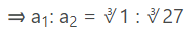⇒ a1 : a2 = 1 : 3

⇒ (a1)2 : (a2)2 = 12: 32

⇒ (a1)2 : (a2)2 = 1 : 9

∴ Ratio of the surface areas of the given cubes is 1 : 9.

48. The diameter of the base of a cylinder is 4 cm and its height is 14 cm. The volume of the cylinder is

A. 176 cm3

B. 196 cm3

C. 276 cm3

D. 352 cm3

Solution

Given: Diameter of the base of a cylinder is 4 cm.

Its height is 14 cm.

Volume of the cylinder is given by: πr2h. (here r and h are radius and diameter respectively)

Let V be the volume of the cylinder.

∴ V = πr2h

⇒ V = π × (4/2)2 × 14 (∵ 4 is the diameter and 4/2 is the radius)

⇒ V = π × (2)2 × 14 = π × 4 × 14

⇒ V = 176 cm3

∴ The volume of the cylinder is 176 cm3

49. The diameter of a cylinder is 28 cm and its height is 20 cm. The total surface area of the cylinder is

A. 2993 cm2

B. 2992 cm2

C. 2292 cm2

D. 2229 cm2

Solution

Given: Diameter of the base of a cylinder is 28 cm.

Its height is 20 cm.

Volume of the cylinder is given by: 2πr(r + h). (Here r and h are radius and diameter respectively)

Let S be the Surface area of the cylinder.

∴ V = 2πr(r + h)

⇒ S = 2 × π × (28/2) × [(28/2) + 20] (∵ 28 is the diameter and 28/2 is the radius)

⇒ V = 2 × π × 14 × (14 + 20) = 2 × π × 14 × 24

⇒ V = 2992 cm2

∴ The Surface area of the cylinder is 2992 cm2

50. The height of a cylinder is 14 cm and its curved surface area is 264 cm2. The volume of the cylinder is

A. 308 cm3

B. 396 cm3

C. 1232 cm3

D. 1848 cm3

Solution

Given: The height of a cylinder is 14 cm.

Its curved surface area is 264 cm2.

Curved surface area of a cylinder is: 2πrh (here r and h are radius and height respectively)

∴ 2πrh = 264

⇒ 2 × π × r × 14 = 264

⇒ r = 264/(2 × π × 14) = 3 cm

∴ r = 3 cm

Volume of a cylinder is: πr2h

Let V be the volume of the cylinder

∴ V = πr2h

⇒ V = π × 32 × 14 = 396 cm3

∴ Volume of the cylinder is 396 cm3

51. The curved surface area of a cylinder is 1760 cm2 and its base radius is 14 cm. The height of the cylinder is

A. 10 cm

B. 15 cm

C.  20 cm

D. 40 cm

Solution

Solution

Given:

The radius of a cylinder is 14 cm.

Its curved surface area is 1760 cm2.

Curved surface area of a Cylinder is 2πrh (here r and h are radius and height respectively)

∴ 2πrh = 1760

⇒ 2 × π × 14 × h = 1760

⇒ h = 1760/(2 × π × 14) = 20 cm

∴ h = 20 cm

That is height of the given Cylinder is 20 cm.

52. The ratio of the total surface area to the lateral surface area of a cylinder with base radius 80 cm and height 20 cm is

A. 2 : 1

B. 3 : 1

C. 4 : 1

4. 5 : 1

Solution

Given: Radius and height of the cylinder are 80 cm and 20 cm respectively.

Lateral surface area of a Cylinder is: 2πrh

Let S1 be the Lateral surface area of the Cylinder

∴ S1 = 2πrh

⇒ S1 = 2 × π × 80 × 20 = 3200

Total Surface area of a cylinder is 2πr(r + h)

Let S2 be the Total surface area of the cylinder

∴ S2 = 2πr(r + h)

⇒ S2 = 2 × π × 80 × (80 + 20) = 16000π

Ratio of Total surface area to lateral surface area is S2 : S1

∴ S2 : S1 = 16000π : 3200π

⇒ S2 : S1 = 5 : 1

∴ The ratio of the total surface area to the lateral surface area of a cylinder is 5 : 1.

53. The curved surface area of a cylindrical pillar is 264 m2 and its volume is 924 m3. The height of the pillar is

A. 4 m

B. 5 m

C. 6 m

D. 7 m

Solution

Given: CSA of a cylindrical pillar is 264 m2

Volume of cylindrical pillar is 924 m3

CSA of a cylinder is : 2πrh (here r and h are radius and height respectively)

∴ 2πrh = 264 …(i)

Volume of the Cylinder is: πr2h (here r and h are radius and height respectively)

∴ πr2h = 924 …(ii)

Divide (ii) by (i)

We get,

(π × r × r × h)/(2 × π × r × h) = 924/264

⇒ r/2 = 7/2

⇒ r = 7

∴ r = 7

Substitute ‘r’ in (i)

∴ 2 × π × 7 × h = 264

⇒ 2 × 22/7 × 7 × h = 264

⇒ 44 × h = 264

⇒ h = 264/44 = 6 cm

∴ height of the cylindrical pillar is 6 cm.

54. The ratio between the radius of the base and height of the cylinder is 2 : 3. If its volume is 1617 cm3, the total surface area of the cylinder is

A. 308 cm2

B. 462 cm2

C. 540 cm2

D. 770 cm2

Solution

Given: The ratio between the radius of the base and the height of the cylinder is 2 : 3.

Volume of the Cylinder is 1617 cm3

Let 2x and 3x be radius and height of the cylinder respectively.

Volume of the Cylinder is given as: πr2h

∴ πr2h = 1617

⇒ π × (2x)2 × (3x) = 1617

⇒ 12π × x3 = 1617

⇒ x3 = 1617/12π = (1617 × 7)/(22 × 12) = 343/8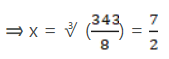∴ r = 2 × 7/2 = 7 cm

And, h = 3 × 7/2 = 10.5 cm

Total surface area of a cylinder is: 2πrh(r + h)

Let S be the TSA of a cylinder

∴ S = 2πrh(r + h)

⇒ S = 2 × π × (7) × (7 + 10.5) = 2 × π × (7) × 17.5 = 770

∴ S = 770 cm2

That is, Total surface area of the cylinder is 770 cm2

55. The radii of two cylinders are in the ratio 2 : 3 and their height in the ratio 5 : 3. The ratio of their volume is

A. 27 : 20

B. 20 : 27

C. 4 : 9

D. 9 : 4

Solution

Given:

The radii of two cylinders are in the ratio 2 : 3.

Heights of the cylinders are in the ratio 5 : 3.

Volume of cylinder is: πr2h (here r and h are radius and height of the cylinder respectively)

Let V1 be the volume of first cylinder

∴ V1 = π(r1)2h1

Let V2 be the volume of second cylinder

∴ V2 = π(r2)2h2

∴ V1 : V2 = π(r1)2h1: π(r2)2h2

⇒ V1 : V2 = π × (2)2 × 5 : π × (3)2 × 3

⇒ V1 : V2 = 20π : 27π = 20 : 27

∴ V1 : V2 = 20 : 27

That is the ratio of their volume is 20 : 27.

56. Two circular cylinder of equal volume have their height in the ratio 1 : 2. The ratio of their radii is

A. 1 : √2

B. √2 : 1

C. 1 : 2

D. 1 : 4

Solution

Given: Two cylinders of equal volume.

Heights of the cylinders are in the ratio 1 : 2.

Volume of cylinder is: πr2h (here r and h are radius and height of the cylinder respectively)

Let V1 be the volume of first cylinder

∴ V1 = π(r1)2h1

Let V2 be the Volume of second cylinder

∴ V2 = π(r2)2h2

Here,

V1 = V2

⇒ π(r1)2h1 = π(r2)2h2

⇒ (r1)2h1 = (r2)2h2

⇒ (r1)2 : (r2)2 = h2 : h1

⇒ (r1)2 : (r2)2 = h2 : h1

⇒ (r1)2 : (r2)2 = 2 : 1

⇒ r1 : r2 = √2 : 1

∴ ratio of the radii of given cylinders is √2 : 1

57. The radius of the base of a cone is 5 cm and its height is 12 cm. Its curved surface area is

A. 60π cm2

B. 65π

C. 30π cm2

D. None of these

Solution

Given: The height of the cone is 12 cm

The radius of the cone is 5 cm.

Curved surface area of a cone is πrl (here r and l are radius and slant height respectively)

l = √(r2 + h2)

∴ πrl = πr√(r2 + h2)

Let S be the CSA of the cone.

∴ S = πr√(r2 + h2)

⇒ S = π × (5) × √(5)2 + (12)2)

⇒ S = π × (5) × √(25 + 144) = π × (5) × √(169)

⇒ S = π × (5) × √(25 + 144) = π × (5) × √(169)

⇒ S = π × (5) × 13 = 65π

∴ S = 65π cm2

That is CSA of the cone is 65π cm2.

58. The diameter of the base of a cone is 42 cm and its volume is 12936 cm3. Its height is

A. 28 cm

B. 21 cm

C. 35 cm

D. 14 cm

Solution

Given: Diameter of the base of a cone is 42 cm.

Volume of the cone is 1293 cm3.

Volume of the cone is given by: 1/3 × π × r2 × h

∴ 1/3 × π × r2 × h = 12936

⇒ 1/3 × π × (42/2)2 × h = 12936 (here diameter = 42 cm, ∴ r = 42/2 = 21)

⇒ 1/3 × π × (21)2 × h = 12936

⇒ h = (12936 × 3)/(π × 21 × 21) = 28 cm

∴ Height of the given cone is 28 cm

59. The area of the base of a right circular cone is 154 cm2 and its height is 14 cm. Its curved surface area is

A. 154√7 cm2

B. 154√7 cm2

C. 77√7 cm2

D. 77√5 cm2

Solution

Given:

Area of the base of a right circular cone is 154 cm2.

Height of the cone is 14 cm.

Curved surface area of a cone is : πrl (here r and l are radius and slant height respectively)

Area of the base is given by : πr2

∴ π × r2 = 154

⇒ r2 = 154/π = 49

⇒ r = √49 = 7 cm

∴ radius of the base of the cone is 7 cm

Now,

l = √(r2 + h2)

∴ πrl = πr√(r2 + h2)

Let S be the CSA of the cone.

∴ S = πr√(r2 + h2)

⇒ S = π × (7) × √(7)2 + (14)2

⇒ S = π × (7) × √(49 + 196) = π × (7) × √(245)

⇒ S = π × (7) × 7√5 = 22/7 × 7√5 = 154√5

∴ S = 154√5 cm2

That is CSA of the cone is 154√5 cm2.

60. On increasing each of the radius of the base and the height of a cone by 20% its volume will be increased by

A. 20%

B. 40%

C. 60%

D. 72.8%

Solution

Given: Radius of the base and the height of a cone is increased by 20%

The volume of the cone is : 1/3πr2h

New radius = r + 20/100.r + 120/100.r = 6/5.r

New volume = 1/3 × π × (6/5r)2 × (6/6.h) = 216/125 × 1/3πr2h

Increase in volume = 216/125 × 1/3πr2h – 1/3πr2h = 91/125 × 1/3πr2h

∴ Increase in % = ((91/125 × 1/3πr2h)/(1/3πr2h) × 100)% = 72.8%

∴ Volume will be increased by 72.8%.

61. The radii of the base of a cylinder and a cone are in the ratio 3 : 4. If they have their height in the ratio 2 : 3, the ratio between their volumes is :

A. 12 cm

B. 14 cm

C. 15 cm

D. 18 cm

Solution

Given: The radii of the base of a cylinder and cone are in the ratio 3 : 4.

Heights of the base of a cylinder and a cone are in the ratio 2 : 3.

Volume of cylinder is : πr2h (here r and h are radius and height of the cylinder respectively)

Volume of cylinder is: πr2h

Let V1 be the volume of first cylinder

∴ V1 = π(r1)2h1

Let V2 be the volume of the cone.

∴ V2 = 1/3π(r2)2h2

∴ V1 : V2 = π(r1)2h1 : 1/3π(r2)2h2

⇒ V1 : V2 = π × (3)2 × 2 : 1/3 × π × (4)2 × 3

⇒ V1 : V2 = 18π : 1/3 × 48π = 18 : 16 = 9 : 8

∴ V1 : V2 = 9 : 8

That is the ratio of their volume is 9 : 8.

62. A metallic Cylinder of radius 8 cm and height 2 cm is melted and converted into aright circular cone of height 6 cm. The radius of the base of the cone is

A. 4 cm

B. 5 cm

C. 6 cm

D. 8 cm

Solution

Given: A metallic Cylinder of radius 8 cm and height 2 cm

Right circular cone of height 6 cm

Volume of a cylinder is given by: π × r2 × h

Volume of a cone is given by : 1/3 × π × r2 × h

Let V1 be the volume of the Cylinder

∴ V1 = π × r2 × h

⇒ V1 = π × (8)2 × (2) = 128π

Let V2 be the volume of the cone

∴ V2 = 1/3 × π × r2 × h

∴ V2 = 1/3 × π × (r)2 × (6) = 2πr2

Here, Solid Cylinder is melted and made into a Solid cone.

∴ V1 : V2

⇒ 128π = 2πr2

⇒ 2πr2 = 128π

⇒ r2 = 128π/2π = 64

⇒ r2 = 64

⇒ r = √64

⇒ r = √64 = 8 cm

∴ Radius of the base of the cone is 8 cm.

63. The height of the conical tent is 14m and its floor area is 346.5 m2. How much canvas, 1.1 m wide, will be required for it.

A. 490 m

B. 525 m

C. 665 m

D. 860 m

Solution

Given:

The height of the conical tent is 14m and its floor area is 346.5 m2.

Canvas of width 1.1 m

Surface area of the tent is: πrl

Let be the Surface area of the cone.

Area of the floor is: πr2 = 346.5 m2

⇒ πr2 = 346.5

⇒ r2 = 346.5/π = 441/4

⇒ r = √(441/4) = 21/2

⇒ l = √(h2 + r2) (here l is the slant height and h, r are height and radius respectively of cone)

⇒ l = √(142 + (21/2)2 = √(196 + 441/4) = √1225/4 = 35/2

∴ S = πrl = π × 21/2 × 35/2 = (33 × 35)/2

∴ Area of the canvas required to cover the tent is: (33 × 35)/2 m2

∴ l × b = (33 × 35)/2 m2 (here l is length and b is breadth or width of the canvas)

⇒ 1 × 1.1 = (33 × 35)/2 (width of the canvas is 1.1 m)

⇒ l = (33 × 35)/(2 × 1.1) = 525 m

∴ Length of the canvas required to cover the tent is 525 m.

64. The diameter of a sphere is 114 cm. Its Volume is

A. 1428 cm3

B. 1439 cm3

C. 1437.1/3 cm3

D. 1440 cm3

Solution

Given: Diameter of the sphere is 14 cm.

Radius of the sphere is 14/2 = 7 cm

Volume of the Sphere is given by: 4/3πr3 (here r is the radius of the sphere)

Let V be the volume of the sphere

∴ V = 4/3.πr3

⇒ V = 4/3.π(7)3 = 4/3 × 22/7 × 343

⇒ V = 4/3 × 22 × 49 = 4312/3 = 1437.1/3

∴ Volume of the sphere is 1437.1/3

65. The ratio between the volumes of two spheres is 8 : 27. What is the ratio between the surface areas?

A. 2 : 3π

B. 4 : 5

C. 5 : 6

D. 4 : 9

Solution

Given Volumes of the spheres are in the ratio 8 : 27.

Volume of a sphere is given by : 4/3πr3 (here ’r’ is the radius of the sphere).

Surface area of a sphere is given by: 4πr2 (here ‘r’ is the radius of the sphere).

Let r1, r2 be the radii of first sphere and second sphere respectively.

∴ 4/3π(r1)3 : 4/3π(r2)3 = 2 : 27

⇒ (r1)3 : (r2)3 = 8 : 27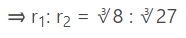⇒ (r1) : (r2) = 2 : 3

Now, here

4π(r1)2 : 4π(r2)2 = (r1)2 : (r1)2

⇒ (r1)2 : (r1)2 = (2)2 : (3)2 = 4 : 9

∴ Ratio of the surface areas of the given spheres is 4 : 9.

66. A hollow metallic Spheres with external diameter 8 cm and internal diameter 4 cm is melted and moulded into a cone having base diameter 8 cm. The height of the cone is

A. 12 cm

B. 14 cm

C. 15 cm

D. 18 cm

Solution

Given: A hollow metallic Sphere with external diameter 8 cm and internal diameter 4 cm

A cone having base diameter 8 cm

Radius of the cone is: 8/2 = 4 cm

External Radius of the sphere is 8/2 = 4 cm

Internal Radius of the sphere is 4/2 = 2 cm

Volume of a Hollow Sphere is given by: 4/3π(r1)3 – (r2)3) (here r1 and r2 are external and Internal radii of the hollow sphere respectively)

Let V1 be the Volume of the Hollow sphere.

∴ V1 = 4/3π(r1)3 - (r2)3)

⇒ V1 = 4/3π(4)3 – (2)3 = 4/3π(64 – 8) = 4/3π(56)

Volume of the cone is given by 1/3 × π × r2 × h

Let  V2 be the volume of the cone

∴ V2 = 1/3 × π × r2 × h

⇒ V2 = 1/3 × π × (4)2 × h

Here, Hollow sphere is melted and moulded into a cone.

∴ V1 = V2

⇒ 4/3.π(56) = 1/3 × π × (8)2 × h

⇒ 1/3 × π × 16 × h = 4/3 × π × (56)

⇒ h = (4/3 × π × 56)/(1/3 × π × 16) = 14 cm

∴ Height of the cone is 14 cm.

67. A metallic cone having base radius 2.1 cm and height 8.4 cm is melted and moulded into a sphere. The radius of the sphere is

A. 2.1 cm

B. 1.05 cm

C. 1.5 cm

D. 2 cm

Solution

Given:

A metallic cone having base radius 2.1 cm and height 8.4 cm

Volume of a cone is given by: 1/3 × π × r2 × h

Let V1 be the volume of the cone

∴ V1 = 1/3 × π × r2 × h

⇒ V1 = 1/3 × π × (2.1)2 × (8.4)

Volume of a sphere is sphere is given by: 4/3 × π × r3

Let V2 be the volume of the cone

∴ V1 = 4/3 × π × r3

Here,

V1 = V2

∴ 1/3 × π × (2.1)2 × (8.4) = 4/3 × π × r3

⇒ r3 = 1/3 × π × (2.1)2 × (8.4) × 3/4π = (2.1)3

⇒ r3 = (2.1)3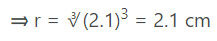∴ Radius of the sphere is 2.1 cm

68. The volume of a hemisphere is 19404 cm3. The total surface area of the hemisphere is

A. 4158 cm2

B. 16632 cm2

C. 8316 cm2

D. none of these

Solution

Given: The volume of a hemisphere is 19404 cm3.

Volume of the hemisphere is given by: 2/3 × π × r3

∴ 2/3 × π × r3 = 19404

⇒ r3 = 19404 × 3/2π = 9261

⇒ r3 = 9261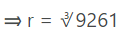⇒ r = 21

Now, Total surface area of hemisphere is given by: 3 πr2

Let S be the TSA

∴ S = 3 × π × r2

⇒ S = 3 × π × (21)2 = 4158 cm2

∴ S = 4158 cm2

That is TSA of the given sphere is 4158 cm2

69. The surface area of a sphere is 154 cm2. The volume of the sphere is

A. 179.2/3 cm3

B. 359.1/3 cm3

C. 1437.1/3 cm3

D. none of these

Solution

Given:

The surface area of a sphere is 154 cm2.

TSA of the sphere is given by: 4 × π × r2

∴ 4 × π × r2 = 154

⇒ r2 = 154 × 1/4π = 49/4

⇒ r2 = 49/4

⇒ r = √49/4

⇒ r = 7/2

Now, Volume of hemisphere is given by: 4/3πr3

Let V be the volume of the hemisphere

∴ V = 4/3 × π × r3

⇒ V = 4/3 × π × (7/2)3 = 539/3 cm3 = 179.2/3 cm3

∴ V = 179.2/3 cm3

That is Volume of the given sphere is 179.2/3 cm3

70. The total surface area of a hemisphere of radius 7 cm is

A. (588π) cm2

B. (329π) cm2

C. (1147π) cm2

D. (98π) cm2

Solution

Given: Radius of the hemisphere: 7 cm

TSA of the hemisphere is given by: 3πr2

Let S be the TSA of the hemisphere.

∴ S = 3πr2

⇒ S = 3 × π × (7)2 = 147π cm2

∴ TSA of the hemisphere is 147π cm2

71. The circular ends of a bucket are of radii 35 cm and 14 cm and the height of the bucket is 40 cm. Its volume is

A. 60060 cm3

B. 80080 cm3

C. 70040 cm3

D. 80160 cm3

Solution

Given: The circular ends of a bucket are of radii 35 cm and 14 cm and the height of the bucket is 40 cm.

Bucket is in the shape of frustum.

Let V be the Volume of the Bucket (Frustum)

Volume of the frustum is given by:π/3 × h × (R2 + r2 + Rr) (here r and R are the radii of smaller and larger circular ends respectively)

∴ V = π/3 × h × (R2 + r2 + R × r)

⇒ V = π/3 × 40 × (352 + 142 + 35 × 14)

⇒ V = π/3 × 40 × (1225 + 196 + 490) = π/3 × 40 × (1911)

⇒ V = π/3 × 40 × (1911) = 80080 cm3

∴ The volume of the bucket is: 80080 cm3

72. If the radii of the end of a bucket are 5 cm and 15 cm and it is 24 cm high then its surface area is (use π = 3.14)

A. 1815.3 cm3

B. 1711.3 cm2

C. 2025.3 cm2

D. 2369 cm2

Solution

Given: The radii of the end of a bucket are 5 cm and 15 cm and it is 24 cm high

Bucket is in the shape of frustum.

TSA of a frustum of a cone = π(r1 + r2) + πr12 + πr22 (here l, r1, r2 are the slant height, radii of the frustum)

Let S be the TSA of the bucket

∴ S = π(r1 + r2) + π(r2)2 (here, top of the bucket is not closed but bottom is closed, ∴ π(r2)2 = 0)

l = √(h2 + (R – r)2)

⇒ S = π × √(h2 + (R – r)2 × (r1 + r2) + π(r2)2

⇒ S = π × √(242 + (15 – 5)2) × (5 + 15) + π × (5)2

⇒ S = π × √(576 + 100) × (20) + π × 25

⇒ S = π × √(676) × (20) × π × 25

⇒ S = π × 520 + π × 25

⇒ S = π × (520 + 25)

⇒ S = 3.14 × 545 = 1711.3 cm2

∴ The surface area of the bucket is 1711.3 cm2.

73. A circus tent is cylindrical to a height of 4 m and conical above it. If its diameter is 105 m and its slant height is 40 m, the total area of canvas required is

A. 1760 m2

B. 2640 m2

C. 3960 m2

D. 7920 m2

Solution

Given: A circus tent is cylindrical to a height of 4 m and conical above it.

Its diameter is 105 m and its slant height is 40 m.

CSA of the Cylinder is given by: 2πrh (here, r and h are radius and height respectively)

Let V1 be the CSA of the cylindrical part of tent

∴ V1 = 2πrh

⇒ V1 = 2 × π × 105/2 × 4 = 1320 cm2 (here diameter is 105 cm, ∴ radius = 105/2 cm)

CSA of the cone is given by: πrl (here, r and l are radius and slant height respectively)

Let V2 be the CSA of the conical part of tent

∴ V2 = πrl

⇒ V2 = π × 105/2 × 40 = 6600 cm2 (here diameter is 105 cm, ∴ radius = 105/2 cm)

∴ Total area of the canvas is V1 + V2

∴ V1 + V2 = 1320 + 6600 = 7920 cm2

∴ Total area of the canvas is: 7920 cm2

74. Match the following columns: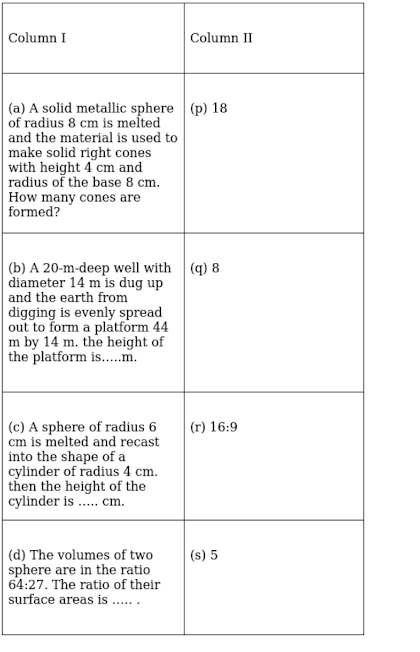Solution

a – (q), b – (s), c – (p), d – (r)

(a) Given:

A solid metallic sphere of radius 8 cm

Solid right cones with height 4 cm and radius of the base 8 cm.

Volume of a metallic sphere is given by: 4/3 × π × r3

Volume of a Right cone is given by: 1/3 × π × r2 × h

Let V1 be the volume of the metallic sphere.

∴ V1 = 4/3 × π × r3 = 4/3 × π × (8)3

Let V2 be the volume of the Solid right cone.

∴ V2 = 1/3 × π × r2 × h = 1/3 × π × (8)2 × 4

Let ‘n’ be the number of right circular cones that are made from melting the metallic sphere.

∴ V1 = n × V2

4/3 × π × (8)3 = n × 1/3 × π × (8)2 × 4

n = (4/3 × π × 8 × 8 × 8)/(1/3 × π × 8 × 8 × 4) = 8

∴ 8 cones are formed from melting the metallic sphere.

(b) Given:

A 20 m deep well with diameter 14 m

A platform 44 m by 14 m

Volume of a cylinder is given: π × r2 × h

Volume of a platform (cuboid) is given by: l × b × h (here l, b, h are length, breadth, height respectively)

Let V1 be the Volume of the well

∴ V1 = π × r2 × h = π × (7)2 × 20

Let V2 be the Volume of the platform

∴ V2 = l × b × h = 44 × 14 × h

Here V1 = V2

∴ 44 × 14 × h = π × (7)2 × 20

⇒ h = (π × 7 × 7 × 20)/(44 × 14) = 5 cm

∴ h = 5 cm

That is height of the platform is 5 cm.

(c) Given:

A sphere of radius 6 cm

A cylinder of radius 4 cm

Volume of a metallic sphere is given by: 4/3 × π × r3

Volume of a cylinder is given by: π × r2 × h

Let V1 be the Volume of the metallic sphere.

∴ V1 = 4/3 × π × r3 = 4/3 × π × (6)3

Let V2 be the Volume of the Solid Cylinder.

∴ V2 = π × r2 × h = π × (4)2 × h

Here V1 = V2

∴ π × (4)2 × h = 4/3 × π × (6)3

⇒ h = (4/3 × π × 6 × 6 × 6)/(π × 4 × 4) = 18 cm

∴ h = 18 cm

That is height of the cylinder is 18 cm.

(d) Given :

Volume ratio of two Spheres is 64: 27

Volume of the Sphere: 4/3 × π × r3 (where r is radius of sphere)

Surface area of the sphere is: 4 × π × r2 (where r is radius of sphere)

Let V1 and V2 be the volumes of different spheres.

V1 : V2 = 64 : 27

4/3 × π × (r1)3 : 4/3 × π × (r2)3 = 64 : 27 (here r1 and r2 are the radii of V1 and V2 respectively)

(r1)3 : (r2)3 = 64 : 27

r1 : r2 = 4 : 3

Now,

Let S1 and S2 be the Surface areas of the spheres.

∴ S1 : S2 = 4 × π × (r1)2 : 4 × π × (r2)2 (here r1 and r2 are the radii of S1 and S2 respectively)

⇒ S1 : S2 = (r1)2 : (r2)2

⇒ S1 : S2 = (4)2 : (3)2

⇒ S1 : S2 = 16 : 9

∴ The ratios of the Surface areas is 16 : 9

75. Match the following columns: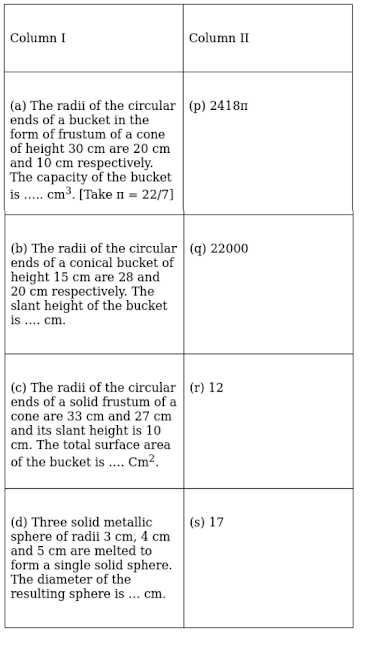Solution

a – (q), b – (s), c – (p), d – (r)

(a) Given:

The radii of the circular ends of a bucket are 20 cm and 10 cm respectively.

Height of the bucket is 30 cm

Bucket is in the shape of frustum.

Let V be the volume of the Bucket (Frustum)

Volume of the frustum is given by: π/3 × h × (R2 + r2 + Rr) (here r and R are the radii of smaller

∴ V = π/3 × h × (R2 + r2 + R × r)

⇒ V = π/3 × 30 × (202 + 102 + 20 × 10)

⇒ V = π/3 × 30 × (400 + 100 + 200) = π/3 × 30 × (700)

⇒ V = π/3 × 30 × (700) = 22000 cm3

∴ The capacity of the bucket is: 22000 cm3

(b) Given:

Height of the frustum of a cone: 15 cm

radii of Circular ends: 28 cm and 20 cm.

Here slant height h can be found by Using Pythagoras theorem.

∴ s2 = h2 + (R – r)2 (here R is 28 cm and r is 20 cm)

⇒ s2 = 152 + (28 – 20)2

⇒ s2 = 152 + (8)2

⇒ s2 = 225 + 64

⇒ s2 = 289

⇒ s = √289 = 17

∴ Slant height of the Frustum is 17 cm

(c) Given:

The radii of the end of a bucket are 33 cm and 27 cm and its slant height is 10 cm

TSA of a frustum of a cone = π(r1 + r2) + πr12 + πr22 (here l, r1, r2 are the slant height, radii of the frustum)

Let S be the TSA of the Frustum.

∴ S = πl(r1 + r2) + π(r2)2 + π(r1)2

⇒ S = π × 10 × (33 + 27) + π(33)2 + π(27)2

⇒ S = π(600 + 1089 + 729 = 2418π

∴ TSA of frustum is 2418π.

(d) Given:

Three solid metallic sphere of radii 3 cm, 4 cm and 5 cm.

Volume of the Solid sphere is: 4/3πr3 (here r is the radius of the sphere).

Let V1 be the volume of the sphere with radius 3 cm.

Let V2 be the volume of the sphere with radius 4 cm.

Let V3 be the volume of the sphere with radius 5 cm.

∴ V1 = 4/3πr3 = 4/3π(3)3

∴ V2 = 4/3πr3 = 4/3π(4)3

∴ V3 = 4/3πr3 = 4/3π(5)3

Here,

Let V be the Volume of the sphere that is formed by melting the spheres with volumes V1, V2, V3.

∴ V = V1 + V2 + V3 = 4/3π(3)3 + 4/3π(3)3 + 4/3π(3)3

⇒ V = 4/3π(33 + 43 + 53) = 4/3π(27 + 64 + 125) = 4/3π(216)

⇒ V = 4/3π(216) = 4/3π(6)3

Here, we can see that radius of the Sphere is 6 cm,

∴ Diameter = 2 × 6 = 12 cm

∴ Diameter of sphere formed by melting spheres with volumes V1, V2, V3 is 12 cm.

76. Each question consists of two statements, namely, Assertion (A) and Reason (R). For selecting the correct answer, use the following code:

Assertion (A): If the radii of the circular ends of a bucket 24 cm high are 15 cm and 5 cm respectively, then the surface area of the bucket is 545π cm2.

Reason (R): If the radii of the circular ends of the frustum of a cone area R and r respectively and its height is h, then its surface area is π{R2 + r2 + l(R – r)}, where l2 = h2 + (R – r)2.

Both Assertion (A) and Reason (R) are true and Reason (R) is a correct explanation of Assertion (A).

Both Assertion (A) and Reason (R) are true and Reason (R) is not a correct explanation of Assertion (A).

Assertion (A) is true and Reason (R) is false.

Assertion (A) is false and Reason (R) is true.

Solution

Assertion is wrong and reason is Wrong.

Explanation:

Assertion (A):

Given: The radii of the end of a bucket are 5 cm and 15 cm and it is 24 cm high.

Bucket is in the shape of frustum.

TSA of a frustum of a cone = πl(r1 + r2) + πr12 + πr22 (here l, r1, r2 are the slant height, radii of the frustum)

Let S be the TSA of the bucket.

∴ S = πl(r1 + r2) + π(r2)2 (here, top of the bucket is not closed but bottom is closed, ∴ π(r2)2 = 0)

l = √(h2 + (R – r)2)

⇒ S = π × √(h2 + (R – r)2) × (r1 + r2) + π(r2)2

⇒ S = π × √(242 + (15 – 5)2 × (5 + 15) + π × (5)2

⇒ S = π × √(576 + 100) × (20) + π × 25

⇒ S = π × √(676) × (20) + π × 25

⇒ S = π × 26 × (20) + π × 25

⇒ S = π × 520 + π × 25

⇒ S = π × (520 + 25)

⇒ S = 3.14 × 545 = 1711.3 cm2

∴ The surface area of the bucket is 1711.3 cm2.

Reason(R):

Here,

Surface area is π {R2 + r2 + l(R + r)}, where l2 = h2 + (R – r)2.

Assertion is wrong and Reason is Wrong.

77. Each question consists of two statements, namely, Assertion (A) and reason (R). For selecting the correct answer, use the following code:

Assertion (A): A hemisphere of radius 7 cm is to be painted outside on the surface. The total cost of painting at Rs per cm2 is Rs 2300.

Reason (R): The total surface area of a hemisphere is 3πr2.

Both Assertion (A) and Reason (R) are true and Reason (R) is a correct explanation of Assertion (A).

Both Assertion (A) and reason (R) are true but reason (R) is not a correct explanation of Assertion (A).

Assertion (A) is true and Reason (R) is false.

Assertion (A) is false and Reason (R) is true.

Solution

Assertion is wrong and Reason is correct.

Explanation:

Assertion (A):

Given: A hemisphere of radius 7 cm.

Total surface area of the hemisphere is: 3πr2

Let S be the TSA of the hemisphere.

∴ S = 3πr2 = 3π(7)2

∴ S = 462 cm2

The total cost to pain it: 462 × 5 = Rs 2310

Reason (R):

The total surface area of a hemisphere is 3πr2.

∴ Assertion is wrong and Reason is correct.

78. Each question consists of two statements, namely, Assertion (A) and Reason (R). For selection the correct answer, use the following code:

Assertion (A) : The number of coins 1.75 cm in diameter and 2 mm thick from a melted cuboid (10 cm × 5.5 cm × 3.5 cm) is 400.

Reason (R): Volume of a cylinder of base radius r and height h is given by V = (πr2h) cubic units. And, area of a cuboid = (l × b × h) cubic units.

Both Assertion (A) and Reason (R) are true and Reason (R) is a correct explanation of Assertion (A).

Both Assertion (A) and Reason (R) are true but Reason (R) is not a correct explanation of Assertion (A).

Assertion (A) is true and Reason (R) is false.

Assertion (A) is false and Reason (R) is true.

Solution

Assertion is correct and Reason is correct explanation of the given assertion.

Explanation:

Assertion (A):

Given: A coin which is 1.75 cm in diameter and 2 mm thick.

A cuboid with dimensions (10 cm × 5.5 cm × 3.5 cm)

A coin is in the form of a cylinder.

Volume of the Cylinder is given by: πr2h

Let V1 be the volume of the coin.

∴ V1 = πr2h

⇒ V1 = π × (1.75/2)2 × 2/10 (here, 1.75 is the diameter, ∴ radius = 1.75/2, and 1 mm = 1/10 cm)

⇒ V1 = π × (0.875)2 × 0.2 = 77/160 cm2

Volume of a cuboid is given by: l × b × h

Let V2 be the volume of the cuboid.

∴ V2 = l × b × h

⇒ V2 = 10 × 5.5 × 3.5 = 192.5 cm2

Let ‘n’ ne the number of coin melted.

∴ n × V1 = V2

⇒ n × 77/160 = 192.5

⇒ n = 192.5 × 160/77 = 400

∴ n = 400

That is 400 coin when melted can be moulded into a cuboid of given dimensions.

Reason(R):

Volume of a cylinder of base radius r and height h is given by V = (πr2h) cubic units. And, area of a cuboid = (l × b × h) cubic units.

∴ Assertion is correct and Reason is correct explanation of the given assertion.

79. Each question consists of two statements, namely, Assertion (A) and Reason (R). For selecting the correct answer, use the following code:

Assertion (A): If the volumes of two sphere are in the ratio 27 : 8 then their surface areas are in the ratio 3 : 2.

Reason (R): Volume of a sphere 4/5πr3.

Surface area of a sphere = 4πR2.

A. Both Assertion (A) and Reason (R) are true and Reason (R) is a correct explanation of Assertion (A).

B. Both Assertion (A) and Reason (R) area true but Reason (R) is not a correct explanation of Assertion (A).

C. Assertion (A) is true and Reason (R) is false.

A. Assertion (A) is false and Reason (R) is true.

Solution

Assertion is wrong and Reason is Wrong.

Explanation:

Assertion (A):

Given: Volumes of two sphere are in the ratio 27 : 8.

Volume of the sphere is given by: 4/3πr3

Let V1 be the volume of the first sphere.

Let V2 be the volume of the first sphere.

∴ V1 : V2 = 4/3π(r1)3 : 4/3π(r2)3

⇒ 27 : 8 = (r1)3 : (r2)3

⇒ r1 : r2 = 3 : 2

Surface area of the sphere is given by: 4πr2

Let S1 be the Surface area of the sphere.

Let S2 be the Surface area of the sphere.

∴ S1 : S2 = 4π(r1)2 : 4π(r2)2

⇒ S1 : S2 = (r1)2 : (r2)2

⇒ S1 : S2 = (3)2 : (2)2

⇒ S1 : S2 = 9 : 4

Reason (R):

Volume of a sphere = 4/3πr3.

Surface area of a sphere = 4πR2.

Assertion is wrong and Reason is Wrong.

80. Each question consists of two statements, namely, Assertion (A) and Reason (R). For selecting the correct answer, use the following code:

Assertion (A): The curved surface area of a cone of base radius 3 cm and height 4 cm is (15π) cm2.

Reason (R) : Volume of a cone = πr2h.

A. Both Assertion (A) and Reason (R) are true Reason (R) is a correct explanation of Assertion (A).

B. Both Assertion (A) and Reason (R) are true but Reason (R) is not a correct explanation of Assertion (A).

C. Assertion (A) is true and Reason (R) is false.

D. Assertion (A) is false and Reason (R) is true.

Solution

Assertion is correct and Reason is Wrong.

Explanation:

Assertion (A):

Given: A cone of radius 3 cm and height 4 cm.

CSA of the cone is given by: πrl (here r is radius and l is slant height)

l = √(h2 + r2)

Let S be the CSA of the cone

∴ S = πrl = π × r × √(h2 + r2)

⇒ S = π × 4 × √(42 + 32) = π × 3 × √(16 + 9) = π × 3 × √(25) = π × 3 × 5 = 15π

∴ S = 15π

Reason (R):

Volume of a cone is: 1/3πr2h

∴ Assertion is correct and Reason is Wrong.

#### Formative Assessment (unit Test)

1. Find the number of solid sphere, each of diameter 6 cm, that could be moulded to

Form a solid metallic cylinder of height 45 cm and diameter 4 cm.

Solution

Given: Diameter of each solid sphere = 6 cm

∴ Radius of each sphere = 6/2 = rs = 3 cm

Diameter of cylinder = 4 cm

∴ Radius of cylinder = 4/2 = rc = 2 cm

Height of cylinder = h = 45 cm

Formula: Volume of sphere = (4/3) × π × rs3

Volume of cylinder = π × rc2 × h

Spheres are moulded to form cylinder which means the volume remains the same

Let ‘n’ be the number of spheres required

i.e., volume of n spheres = volume of cylinder

∴ n × (4/3) × π × 33 = π × 22 × 45

n × 4 × 27 = 4 × 3 × 45

n = 45/9 = 5

Number of solid spheres made = 5

2. Two right circular cylinder of equal volume have their height in the ratio 1 : 2. What is the ratio of their radii ?

Solution

Let the two cylinders be with volume V1 and V2 with their respective radii and height as r1, r2 and h1, h2

Now given ratio of their heights i.e., h1 : h2 = 1 : 2

∴ h1/h2 = 1/2

Volume of cylinder = πr2h

Given that volumes of both cylinder are equal i.e., V1 = V2.

∴ π × r12 × h1 = π × r22 × h2

h1/h2 = r22/r12

r22/r12 = 1/2

r2/r1 = 1/√2

r1/r2 = √2/1

Therefore the ratio of their radii is r1 : r2 = √2 : 1

3. A circus tent is cylindrical to a height of 4 m and conical above it. If its diameter is 105 m and its slant height is 40 m, find the total area of the canvas required.

Solution

Given: Diameter of base of cone and the cylinder = 105 m

∴ Radius of cylinder = rcl = 105/2 = 51 m

Radius of cone = rco = 105/2 = 51 m

Height of cylinder = h = 4 m

Slant height of cone = l = 40 m

Formula: Surface area of cylinder = 2πrclh + 2πrcl2

Surface area of cone = πrco2 + πrcol

Since we don’t require canvas for the top surface and bottom surface of cylinder and also for the base of cone we should subtract those areas from the surface area

Area of upper and lower surfaces of cylinder = 2πrcl2

∴ Area of canvas required for cylinder = 2πrclh + 2πrcl2 - 2πrcl2

= 2πrclh

= 2 × 3.14 × 51 × 4

= 1281.12 m2

Area of base of cone = πrco2

∴ Area of canvas required for cone = πrco2 + πrcol – πrco2

= πrcol

= 3.14 × 51 × 40

= 6405.6 m2

Total area of canvas required = Area of canvas required for cylinder + Area of canvas

Required for cone

= 1281.12 + 6405.6

= 7686.72 m2

∴ Total area of canvas required = 7686.72 m2

4. The radii of the top and bottom of a bucket of slant height 45 cm are 28 cm and 7 cm respectively. Find the curved surface area of the bucket.

Solution

Given: Slant height of bucket = l = 45 cm

Radius of bottom circle = r = 7 cm

Radius of top circle = R = 28 cm

As the bucket is in the form of frustum

Formula: Total surface area of frustum = πr2 + πR2 + π(R + r)l cm2

Now we have asked curved surface area, so we should subtract the top and bottom surface.

Areas which are flat circles.

Surface area of top = πr2

Surface area of bottom = πR2

∴ Curved surface area = total surface area – πr2 – πR2 cm2

= πr2 + πR2 + π(R + r)l – πr2 – πR2 cm2

= π(R + r)l cm2

= 3.14 × (28 + 7) × 45 cm2

= 3.14 × 35 × 45 cm2

= 4945.5 cm2

Therefore, curved surface area of bucket = 4945.5 cm2

5. A solid metal cone with radius of base 12 cm and height 24 cm is melted to form solid spherical balls of diameter 6 cm each. Find the number of balls formed.

Solution

Given: base radius of cone = rc = 12 cm

Height of cone = h = 24 cm

Diameter of spherical ball = 6 cm

Radius of spherical ball: rs = 6/2 = 3 cm

Formula: Volume of cone = (1/3) πrc2h

Volume of sphere = (4/3) πrs3

Let n be the number of spherical balls made

As the cone is melted and then the spherical balls are made therefore the volume remains same

i.e., volume of n spherical balls made = volume of cone

∴ n × (4/3) × π × rs3 = (1/3) × π × rc2 × h

⇒ n × 4 × 33 = 122 × 24

⇒ n × 9 = 12 × 24

⇒ n = 32

∴ Number of balls formed = 32

6. A hemisphere bowl of internal diameter 30 cm is full of a liquid. This liquid is filled into cylindrical – shapes bottles each of diameter 5 cm and height 6 cm. How many bottles are required ?

Solution

Given: Diameter of hemisphere = 30 cm

∴ Radius of hemisphere = rh = 30/2 = 15 cm

Diameter of cylindrical shaped bottles = 5 cm

∴ radius of cylindrical shaped bottles = 5/2 = rc = 2.5 cm

Height of cylindrical shaped bottle = h = 6 cm

Formula: Volume of hemisphere = (Volume of sphere/2) = (2/3)πrh3

Volume of cylinder = πrc2h

Let ‘n’ bottles are required

As we are filling the cylindrical bottles with liquid in hemispherical bowl hence we can say that

Volume of liquid in cylindrical bottles = volume of liquid in hemisphere

∴ n × π × rc2 × h = (2/3) × π × rh3

⇒ n × 2.52 × 6 × 3 = 2 × 153

⇒ n × 6.25 × 9 = 3375

⇒ n = 3375/56.25

⇒ n = 60

Therefore 60 cylindrical shaped bottles are required to fill the liquid from hemispherical bowl.

7. A solid metallic sphere of diameter 21 cm is melted and recast into small cones, each of diameter 3.5 cm and height 3 cm. Find the number of cones so formed.

Solution

Given: Diameter of sphere = 21 cm

∴ radius of sphere = rs = (21/2) cm

Diameter of cone = 3.5 cm

∴ radius of cone = rc = (3.5)/2 = 17.5 = (7/4) cm

Height of cone = h = 3 cm

Formula: Volume of sphere = (4/3)πrs3

Volume of cone = (1/3)πrc2h

Sphere is melted and then cones are made from molten metal therefore the volume remains same

Let ‘n’ be the number of cones made

i.e., volume of n cones = volume of sphere

∴ n × (1/3) × π × rc2 × h = (4/3) × π × rs3

∴ n × 72/42 × 3 = 4 × 213/23

⇒ n = (16 × 21 × 9)/(2 × 3) = 8 × 21 × 3 = 504

Therefore number of cones formed = 504

8. The diameter of a sphere is 42 cm. It is melted and drawn into a cylindrical wire of diameter 2.8 cm. Find the length of the wire.

Solution

Given: Diameter of sphere = 42 cm

∴ Radius of sphere = 42/2 = rs = 21 cm

Diameter of cylindrical wire = 2.8 cm

∴ Radius of cylindrical wire = rc = 1.4 = (7/5) cm

Let l be the length of wire

Formula: Volume of sphere = (4/3)πrs3

Volume of wire = πrc2l

Sphere is melted and wire is made from it

∴ Volume of sphere = Volume of wire

(4/3)πrs3 = πrc2l

⇒ 4 × 213 = 3 × (7/5)2 × l

⇒ 4 × 21 × 9 × 25 = 3 × l

⇒ 2100 × 3 = l

⇒ l = 6300 cm

Therefore length of wire formed = 6300 cm = 63 metres

9. A drinking glass is in the shape of frustum of a cone of height 21 cm with 6 cm and 4 cm as the diameters of its two circular ends. Find the capacity of the glass.

Solution

Given:

Height of glass = h = 21 cm

Diameter of lower circular end of glass = 4 cm

Diameter of upper circular end of glass = 6 cm

∴ Radius of lower circular end = r = 4/2 = 2 cm

∴ Radius of upper circular end = R = 6/2 = 3 cm

Formula: Volume of frustum of cone = 1/3πh(R2 + r2 + Rr) cm3

Capacity of glass = Volume of frustum

∴ Capacity of glass = 1/3 × 22/7 × 21 × (32 + 22 + 3 × 2)

= 22 × (9 + 4 + 6)

= 22 × 19

= 418 cm3

∴ Capacity of glass = 418 cm3

10. Two cubes, each of volume 64 cm3, are joined end to end. Find the total surface area of the resulting cuboid.

Solution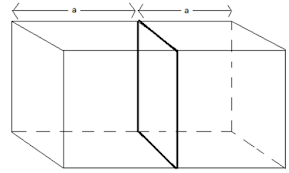Volume of each cube = 64 cm3

Let a be the side length of each cube

∴ a3 = 64

⇒ a = 4 cm

The figure shows both the cubes joined together after joining we get a cuboid of length 2a and breadth a and height a

Length of cuboid formed = l = 2a

l = 8 cm

Breadth of cuboid formed = b = 4 cm

Height of cuboid formed = h = 4 cm

In the cuboid so formed there are 4 rectangular surfaces of length l = 8 and breadth b = 4 and 2 surfaces of length 4

Total surface area of cuboid = 4 × l × b + 2a2

= (4 × 8 × 4) + (2 × 42)

= 128 + 32

= 160 cm2

Total surface area of cuboid = 160 cm2

11. The radius of the base and height of a solid right circular cylinder are in the ratio 2 : 3 and its volume is 1617 cm3. Find the total surface area of the cylinder. [Take π = 22/7]

Solution

Given: Volume of cylinder = 1617 cm3

Let r be the radius of base and h be the height of cone

r : h = 2 : 3

∴ r/h = 2/3

⇒ 3r = 2h

⇒ h = 3r/2 …(i)

Formula: Volume of cylinder = πr2h

∴ 1617 = (22/7) r2h

r2h = 514.5

Using (i), we have

∴ r2 × (3r/2) = 514.5

⇒ 3r3 = 1029

⇒ r3 = 343

⇒ r = 7 cm

h = 21/2 cm

Total surface area of cylinder = 2πr2 + 2πrh

= 2 × (22/7) × 72 + 2 × (22/7) × 7 × (21/2)

= 308 + 462

= 770 cm2

Therefore total surface area of cylinder = 770 cm2

12. A toy is in the form of a cone mounted on a hemisphere on a hemisphere of common base radius 7 cm. The total height of the toy is 31 cm. Find the total surface area of the toy.

Solution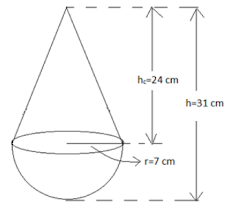Given: Height of toy = h = 31 cm

Radius of hemisphere = radius of base of cone = r = 7 cm

From the figure we can calculate height of cone as

Height of cone hc = h – r

= 31 – 7 = 24 cm

∴ hc = 24 cm

Formula: Surface area of hemisphere = surface area of sphere/2 = 2πr2

Curved surface area of cone = πrl

Where l is slant height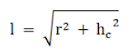⇒ l = √(49 + 576) = 25 cm

∴ l = 25 cm

Total surface area of toy = curved surface area of cone + surface area of hemisphere

Surface area of hemisphere = 2 × π × r2

= 2 × (22/7) × 72

= 308 cm2

Curved surface area of cone = π × r × l

= (22/7) × 7 × 25

= 550 cm2

∴ Total surface area of toy 308 + 550

= 858 cm2

∴ Total surface area of toy = 858 cm2

13. A hemispherical bowl of internal radius 9 cm is full of water. This water is to be filled in cylindrical bottles of diameter 3 cm and height 4 cm. Find the number of bottles needed to fill the whole water of the bowl.

Solution

Given: Radius of hemisphere = rh = 9 cm

Diameter of cylindrical shaped bottles = 3 cm

∴ Radius of cylindrical shaped bottles = rc = 3/2 = 1.5 cm

Height of cylindrical shaped bottle = h = 4 cm

Formula: Volume of hemisphere = (Volume of sphere/2) = (2/3)πrh3

Volume of cylinder = πrc2h

Let ‘n’ bottles are required

As we are filling the cylindrical bottles with liquid in hemispherical bowl hence we can say that

Volume of liquid in cylindrical bottles = volume of liquid in hemisphere

∴ n × π × rc2 × h = (2/3) × π × rh3

⇒ n × (3/2)2 × 4 × 3 = 2 × 93

⇒ n = 33 × 2

⇒ n = 27 × 2

⇒ n = 54

Therefore 54 cylindrical shaped bottles are required to fill the liquid from hemispherical bowl.

14. The surfaces areas of a sphere and a cube are equal. Find the ratio of their volumes. [Takes π = 22/7]

Solution

Let r be the radius of sphere and a be the side length of cube.

Let Ss be the surface area of sphere and Sc be the surface area of cube and Vs be the volume of sphere and Vc be the volume of cube

∴ Ss = 4πr2 and Sc = 4a2

Given that surface area of sphere and cube are equal

∴ Ss = Sc

4πr2 = 6a2

r2/a2 = 3/2π

∴ r/a = √3/√2π …(i)

Vs = (4/3) πr3

⇒ Vc = a3

∴ Vs/Vc = 4πr3/3a3

Using (i)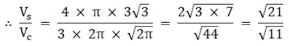∴ Vs/Vc = (√21)/(√11)

Therefore ratio of their volumes is Vs: Vc = √21 : √11

15. The slant height of the frustum of a cone is 4 cm and the perimeters (i.e., circumferences) of its circular ends area 18 cm and 6 cm. Find the curved surface area of the frustum.

Solution

Given: Perimeter of upper circle = 18 cm

Perimeter of lower circle = 6 cm

Slant height of frustum = l = 4 cm

Formula: Total surface area of frustum = πr2 + πR2 + π(R + r)l cm2

Let r be the radius of lower circle and R be the radius of upper circle

Now perimeter of circle = Circumference of circle = 2π × radius

∴ Perimeter of upper circle = 2πR

18 = 2 × π × R

⇒ R = 9/π cm

Perimeter of lower circle = 2πr

6 = 2 × π × r

⇒ r = 3/ π cm

Now we have asked curved surface area, so we should subtract the top and bottom surface areas which are flat circles.

Surface area of top = πR2

Surface area of bottom = πr2

∴ Curved surface area = total surface area – πr2 – πR2 cm2

= πr2 + πR2 + π(R + r)l – πr2 - πR2 cm2

= π(R + r)l cm2

= π × [(9/π) + (3/π) × 4 cm2

= (9 + 3) × 4 cm2

= 48 cm2

∴ Curved surface area = 48 cm2

16. A solid is composed of a cylinder with hemisphere ends. If the whole length of the solid is 104 cm and the radius of each hemispherical and is 7 cm, find the surface area of the solid.

Solution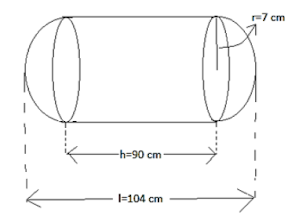Total length of solid = l = 104 cm as shown in figure

The solid consist of a cylinder and two hemispheres

Let the height of cylinder be h

We get the height h by subtracting the radii of left and right hemisphere from the total length l as seen in figure

∴ h = 104 – (7 + 7) cm

∴ h = 90 cm

Let r be the radius of hemisphere and the radius of cylinder

∴ r = 7 cm

There are two hemisphere one at left and one at right both of same radius r and two hemispheres make one sphere

Surface area of sphere = 4πr2

The flat circles i.e., the upper and lower circles of cylinder are not to be considered in the surface area of whole solid as they are covered by the hemisphere therefore for cylinder we will take its curved surface area

Curved surface area of cylinder= 2πrh

Surface area of solid = surface area of sphere + curved surface area of cylinder

= 4πr2 + 2πrh

= 2 × (22/7) × 7 × (2 × 7 + 90)

= 44 × 104

= 4576 cm2

Therefore total surface area of solid = 4576 cm2

17. From a solid cylinder whose height is 15 cm and diameter 16 cm, a conical cavity of the same height and the same diameter is hollowed out. Find the total surface area of the remaining solid. [Use π = 3.14]

Solution

After removing the conical solid the cylinder would look like this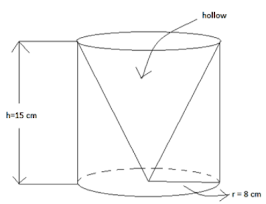Given: height of cylinder = height of cone = h = 15 cm

Diameter of cylinder = diameter of base cone = 16 cm

∴ radius of cylinder = radius of base of cone = 16/2 = r = 8 cm

Formula: total surface area of cylinder = 2πr2h + πrh

Total surface area of cone = πrl + πr2

Where l is the slant height

l = √(r2 + h2)

⇒ l = √(82 + 152)

⇒ l = √289

⇒ l = 17 cm

In the solid as seem in figure we have the curved surface of cylinder and the base of cylinders as there is no top circular face of the cylinder we should subtract its area from that surface area of cylinder

Area of top circular surface of cylinder = πr2

∴ surface area of cylinder in solid = 2πr2 + πrh – πr2

= πr2 – πrh

= 3.14 × 8 × (8 + 15)

= 3.14 × 8 × 23

= 577.76 cm2

Now there is a hollow conical part with no base of the cone as seen in the figure therefore we should subtract the surface area of base of cone from the total surface area of cone.

Surface area of base of cone = πr2

∴ Surface area of conical part in solid = πrl + πr2 - πr2

= πrl

= 3.14 × 8 × 17

= 427.04 cm2

Therefore total surface area of solid = Surface area of cylinder in solid + surface area of conical part in solid

= 577.76 + 427.04

= 1004.8 cm2

Surface area of solid = 100.8 cm2

18. A solid rectangular block of dimension 4.4 m, 2.6 m, 1 m is cast into hollow cylindrical pipe of internal radius 30 cm and thickness 5 cm. Find the length of the pipe.

Solution

Given: length of the block = l = 4.4 m

Width of the block = w = 2.6 m

Height of the book = h = 1 m

Inner radius of pipe = r = 30 cm = 0.3 m

Thickness of pipe = t = 5 cm = 0.05 m

∴ outer radius of pipe as seen in the cross section of pipe = R = r + t = 30 + 5 = 35 cm = 0.35 m

Let l be the length f the pipe

Formula: Volume of block = l × w × h

= 4.4 × 2.6 × 1

= 11.44 m3

Volume of block = 11.44 m3

Volume of pipe = π × (radius)2 × (length)

Volume of pipe material = volume of full pipe(R = 0.35) – Volume of hollow cylinder(r = 0.3)

= π × 0.352 × l × π × 0.32 × l

= π × l × [(35/100)2 – (3/10)2]

= π × l × [(35/100) + (3/10)] × [(35/100) – (3/10)]

= π × l × 65/100 × 5/100

= (22/7) × l × (13/400) m3

∴ volume of pipe material = (22/7) × l × (13/400) m3

The pipe is made from the block

∴ volume of block = volume of pipe material

∴ 11.44 = (22/7) × l × (13/400)

∴ l = (7 × 400 × 11.44)/(22 × 13) = (28 × 52)/13

∴ l = 28 ×

∴ l = 112 m

Length of the pipe = 112 m

19. An open metal bucket is in the shape of a frustum of a cone, mounted on a hollow cylindrical base made of the same metallic sheet. The diameter of the two circular ends of the bucket are 45 cm and 25 cm, the total vertical height of the bucket is 40 cm and that of the cylindrical base is 6 cm. Find the area of the metallic sheet used to make the bucket. Also, find the volume of water the bucket cam hold, in litres.

Solution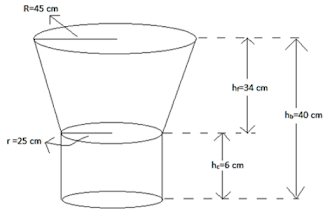Give:

Radius of upper circular end of frustum = R = 45 cm

Radius of lower circular end of frustum = radius of cylindrical base = r = 25 cm

Height of bucket = hb = 40 cm

Height of cylindrical base = hc = 6 cm

From the figure height of frustum = hf = hb – hc

= 40 – 6

∴ hf = 34 cm

Formula: Volume of frustum of cone = 1/3πhf (R2 + r2 + Rr) cm3

Volume of cylinder = πr2hc

Curved surface area of cylinder = 2πrhc

Curved surface area of frustum = π(R + r)l cm2

Where l = slant heightArea of metallic sheet used = curved surface area of frustum + curved surface area of base

Cylinder + area + base circle of cylinder

Now, curved surface area of frustum = π × (R + r) × l cm2

= (22/7) × (45 + 25) × 39.44 cm2

= 22 × 10 × 39.44 cm2

= 8676.8 cm2

Curved surface area of base cylinder = 2πrhc

= 2 × (22/7) × 25 × 6

= 942.85 cm2

Surface area of base circle of cylinder = πr2

= (22/7) × 252

= 1964.28 cm2

∴ Area of metallic sheet used = 8676.8 + 942.85 + 1964.28

= 11583.93 cm2

Therefore, area of metallic sheet used to make the bucket is 11583.93 cm2 i.e., 1.158393 m2

Volume of water bucket can hold = volume of gas cylinder + volume of frustum

Volume of base cylinder = πr2hc

= (22/7) × 252 × 6

= 11785.71 cm3

Volume of frustum = 1/3 × 22/7 × 34 × (452 + 252 + 45 × 25)

= 748/21 × (2025 + 1750)

= 35.62 × 3775

= 134465.5 cm3

∴ Volume of water bucket can hold = 11785.71 + 134465.5

= 146251.21 cm3

Now 1 litre is 1000 cm3

∴ 146251.21 cm3 = (146251.21)/1000 = 146.25121 litres

Volume of water bucket can hold = 146.25121 litres

20. A farmer connect a pipe of internal diameter 20 cm from a canal into a cylindrical tank which is 10 m diameter and 2 m deep. If the water flows through the pipe at the of 4 km/hr, in how much time will the tank be filled completely ?

Solution

Given: diameter of pipe = 20 cm

∴ radius of pipe = rp = 20/2 = 10 cm = 0.1 m

Diameter of tank = 10 m

∴ radius of cylindrical tank = rc = 10/2 = 5 m

Depth of cylindrical tank = height of cylindrical tank = h = 2 m

Rate of flow of water through pipe = 4 km/hr

1 km = 1000 m

4 km/hr = 4000 m/hr

Volume of water required to completely fill the tank is equal to the volume of cylinder

Time require to fill the tank = (volume of cylindrical tank)/(volume of water flown through pipe per hr)

Volume of cylindrical tank = π × rc2 × h

= 3.14 × 25 × 2

= 157 m3

Volume of water flown through pipe per hr = π × rp2 × 4000

= 3.14 × 0.12 × 4000

= 3.14 × 40

= 125.6 m3/hr

Time require to fill the tank = 157/125.6

= 1.25 hrs

Therefore, it will take 1.25 hours to fill the tank completely.